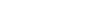How Cheenta works to ensure student success?
Explore the Back-Story

# Number Theory and Geometry | PRMO 2019 | Problem 6Try this beautiful problem from Pre RMO 2019 based on Number Theory and Geometry.

## Number Theory and Geometry - PRMO 2019

Let abc be a three digit number with nonzero digits such that. Find is the largest possible prime factor of abc.

• is 13
• is 29
• is 840
• cannot be determined from the given information

### Key Concepts

Sequence

Geometry

Number Theory

PRMO, 2019

Elementary Number Theory by David Burton

## Try with Hints

Here a,b,c form Pythagoras triplet then abc=345 or 435

345=(3)(5)(23) and 435=(5)(3)(29)

Then largest possible prime factor=29

## Subscribe to Cheenta at Youtube

Try this beautiful problem from Pre RMO 2019 based on Number Theory and Geometry.

## Number Theory and Geometry - PRMO 2019

Let abc be a three digit number with nonzero digits such that. Find is the largest possible prime factor of abc.

• is 13
• is 29
• is 840
• cannot be determined from the given information

### Key Concepts

Sequence

Geometry

Number Theory

PRMO, 2019

Elementary Number Theory by David Burton

## Try with Hints

Here a,b,c form Pythagoras triplet then abc=345 or 435

345=(3)(5)(23) and 435=(5)(3)(29)

Then largest possible prime factor=29

## Subscribe to Cheenta at Youtube

This site uses Akismet to reduce spam. Learn how your comment data is processed.

### Knowledge Partner